# Statistical Process Control

Statistical Process Control is an analytical decision making tool which allows you to see when a process is working correctly and when it is not. Variation is present in any process, deciding when the variation is natural and when it needs correction is the key to quality control. Control charts show the variation in a measurement during the time period that the process is observed. In contrast, bell-curve type charts, such as histograms or process capability charts, show a summary or snapshot of the results. Control charts are an essential tool of continuous quality control. Control charts monitor processes to show how the process is performing and how the process and capabilities are affected by changes to the process. This information is then used to make quality improvements.
Control charts are also used to determine the capability of the process. They can help identify special or assignable causes for factors that impede peak performance. Control charts show if a process is in control or out of control. They show the variance of the output of a process over time, such as a measurement of width, length or temperature. Control charts compare this variance against upper and lower limits to see if it fits within the expected, specific, predictable and normal variation levels. If so, the process is considered in control and the variance between measurements is considered normal random variation that is inherent in the process. If, however, the variance falls outside the limits, or has a run of non-natural points, the process is considered out of control.

The foundation for Statistical Process Control was laid by Dr. Walter Shewart working in the Bell Telephone Laboratories in the 1920s conducting research on methods to improve quality and lower costs. He developed the concept of control with regard to variation, and came up with Statistical Process Control Charts which provide a simple way to determine if the process is in control or not. Dr. W. Edwards Deming built upon Shewart’s work and took the concepts to Japan following WWII. There, Japanese industry adopted the concepts wholeheartedly. The resulting high quality of Japanese products is world-renowned. Dr. Deming is famous throughout Japan as a “God of quality”. Today, SPC is used in manufacturing facilities around the world.

Process control charts are fairly simple-looking connected-point charts. The points are plotted on an x/y axis with the x-axis usually representing time. The plotted points are usually averages of subgroups or ranges of variation between subgroups, and they can also be individual measurements. Some additional horizontal lines representing the average measurement and control limits are drawn across the chart. Notes about the data points and any limit violations can also be displayed on the chart.

### Objectives

Statistical process control (SPC) is a technique for applying statistical analysis to measure, monitor, and control processes. The major component of SPC is the use of control charting methods. The basic assumption made in SPC is that all processes are subject to variation. This variation may be classified as one of two types, chance cause variation and assignable cause variation. Benefits of statistical process control include the ability to monitor a stable process and determine if changes occur, due to factors other than random variation. When assignable cause variation does occur, the statistical analysis facilitates identification of the source . so it can be eliminated. Statistical process control also provides the ability to determine process capability, monitor processes, and identify whether the process is operating as expected, or whether the process has changed and corrective action is required.  Control chart information can be used to determine the natural range of the process, and to compare it with the specified tolerance range. If the natural range is wider, then either the specification range should be expanded, or improvements will be necessary to narrow the natural range. SPC helps us to identify

• Average level of the quality characteristic
• Basic variability of the quality characteristic
• Consistency of performance

Benefits from control charting are derived from both attribute and variable charts. Once the control chart shows that a process is in control, and within specification limits, it is often possible to eliminate costs relating to inspection.  Control charts may be used as a predictive tool to indicate when changes are required in order to prevent the production of out of tolerance material. As an example, in a machining operation, tool wear can cause gradual increases or decreases in a part’s dimension. Observation of a trend in the affected dimension allows the operator to replace the worn tool before defective parts are manufactured. When the manufacturing method is lot production, followed by lot inspection, if inspection finds out of tolerance parts, very little can be done other than to scrap, rework or accept the defective parts. Using control charts, if the process changes, the process can be stopped and only the parts produced since the last check need to be inspected. By monitoring the process during production, if problems do arise, the amount of defective material created is significantly less than when using batch production and subsequent inspection methods. An additional benefit of control charts is the ability to monitor continuous  improvement efforts. When process changes are made which reduce variation, the control chart can be used to determine if the changes were effective. The benefits of statistical process control are not without costs. Costs associated with SPC include selection of the variable(s) or attribute(s) to monitor, setting up the control charts and data collection system, training personnel, and investigating and correcting the cause when data values fall outside control limits. Many companies have found that the benefits of statistical process control far outweigh the related costs.

### The Process

By the process, we mean the whole combination of suppliers, producers, people, equipment, input materials, methods, and environment that work together to produce output, and the customers who use that output. The total performance of the process depends upon communication between supplier and customer, the way the process is designed and implemented , and on the way it is operated and managed. The rest of the process control system is useful only if it contributes either to maintaining a level of excellence or to improving the total performance of the process.

#### Dynamic Processes

A process that is observed across time is known as a dynamic process. An SPC chart for a dynamic process is often referred to as a ‘time-series’ or a ‘longitudinal’ SPC chart.

##### Example of Dynamic process – Coloured beads pulled from a bag

A bag contains 100 beads that are identical – except for colour. Twenty of the beads are red and 80 are blue. Scoopfuls of 20 are repeatedly drawn out, with replacement, and the number of red beads in each scoop is observed.Twenty of the 100 beads in the bag are red, which means that the proportion of red beads in the bag is 1/5. Therefore, if a sample of 20 is drawn each time, we expect four of the beads in the sample to be red, on average. In figure above the plotted points oscillate around four. In general, every time a sample of 20 is drawn you won’t necessarily observe four reds. The number that you observe will vary due to random variation. The random variation that you see in the graph above is common cause variation as there is no unusual behavior in this process. If a sample of 20 beads were drawn from the bag and 10 or more red beads were consistently being observed then this would indicate something unusual in the process i.e. special cause variation which may require further investigation.
The example above is a simplification of Deming’s red bead experiment where the red beads represent an undesired outcome of the process. This process is not dissimilar to the many situations that often occur in healthcare processes. This is how data, which is collected over time, is typically presented and it shows the behavior and evolution of a dynamic process.

#### Static  Processes

A process that is observed at a particular point in time is known as a static process. An SPC chart for a static process is often referred to as a ‘cross-sectional’ SPC chart. A cross-sectional SPC chart is a good way to compare different institutions. For example, hospitals or health boards can be compared as an alternative to league tables

##### Example of Static process – Coloured beads pulled from a bag

There are 10 groups in a room and each group has a bag that contains 20 beads – four of these beads are red. Each group is required to draw out 10 beads and the number of red beads in each groups’ scoop is observed.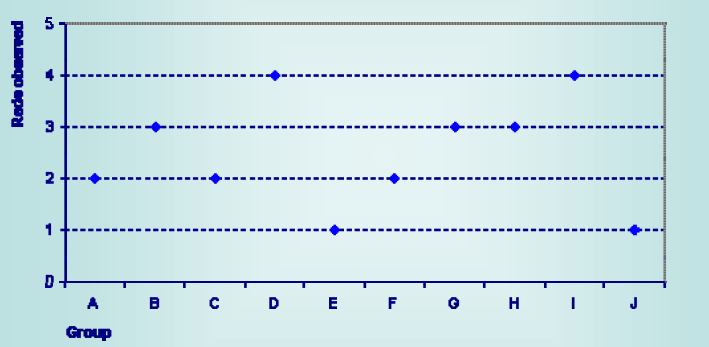The proportion of red beads in the bag is again 1/5. Therefore, if each group draws out a sample of 10, we expect two of the beads in the sample to be red, on average. In figure above the plotted points oscillate around two. The variation in this sample is again random variation (common cause variation). This example illustrates how data is typically presented at a single point in time and it is an example of a static process. This situation arises when data is analyzed across units. This example illustrates how data is typically presented at a single point in time and it is an example of a static process. This situation arises when data is analyzed across units. For example, NHS boards, GP practices, surgical nits etc. and is known as a cross-sectional chart.

### Understanding Variation:

Variation exists in all processes around us. For example:

• Every person is different
• No two snowflakes are identical
• Each fingerprint is unique

Variation in a process can occur through a number of different sources. For example:

• People – Every person is different
•  Materials – Each piece of material/item/tool is unique
•  Methods – Signatures for example
•  Measurement – Samples from certain areas etc can bias results
•  Environment – The effect of seasonality

The two types of variation that we are interested in are ‘common cause’ and ‘special cause’ variation. Variation is the key to statistical process control charts. The extent of variation in a process indicates whether a process is working as it should. When the variation between the points is large enough for the process to be out of control, the variation is determined to be due to non-natural or assignable (special) causes.

#### Common Cause

All processes have random variation – known as ‘common cause variation’. A process is said to be ‘in control’ if it exhibits only common cause variation i.e. the process is completely stable and predictable.

#### Special Cause

Unexpected events/unplanned situations can result in ‘special cause variation’. A process is said to be ‘out of control’ if it exhibits special cause variation i.e. the process is unstable.

SPC charts can be applied to both dynamic processes and static processes. Common cause variation is an inherent part of every process. Generally, the effect of this type of variation is minimal and results from the regular rhythm of the process. Special cause variation is not an inherent part of the process. This type of variation highlights something unusual occurring within the process and is created by factors that were not part of the process’ design. However, these causes are assignable and in most cases can be eliminated. If common cause is the only type of variation that exists in the process then the process is said to be ‘in control’ and stable. It is also predictable within set limits i.e. the probability of any future outcome falling within the limits can be stated approximately. Conversely, if special cause variation exists within the process then the process is described as being ‘out of control’ and unstable.

Shewhart goes on to define control:
“A phenomenon will be said to be controlled when, through the use of past experience, we can predict, at least within limits, how the phenomenon may be expected to vary in the future. Here it is understood that prediction within limits means that we can state, at least approximately, the probability that the observed phenomenon will fall within the given limits.”

The critical point in this definition is that control is not defined as the complete absence of variation. Control is simply a state where all variation is predictable variation. In all forms of prediction there is an element of chance. Any unknown cause of variation is called a chance cause. If the influence of any particular chance cause is very small, and if the number of chance causes of variation are very large and relatively constant, we have a situation where the variation is predictable within limits. For eg we have a box of 100 mangos. if you weighed every single mango you would probably notice that there was a distinct pattern to the weights. In fact, if you drew a small random sample of, say, 25 mango you could probably predict the weights of those mango remaining on the box. This predictability is the essence of a controlled phenomenon. The number of common causes that account for the variation in mango weights is astronomical, but relatively constant. A constant system of common causes results in a controlled phenomenon. Not all phenomena arise from constant systems of common causes. At times, the variation is caused by a source of variation that is not part of the constant system. These sources of variation were called assignable causes by Shewhart; Deming calls them “special causes” of variation. Experience indicates that special causes of variation can usually be found and eliminated. Statistical tools are needed to help us effectively identify the effects of special causes of variation. Statistical process control (SPC) uses of statistical methods to identify the existence of special causes of variation in a process.

The basic rule of statistical process control is:
Variation from common cause systems should be left to chance, but special causes of variation should be identified and eliminated.The figure above illustrates the basic concept. Variation between the control limits designated by the two lines will be considered to be variation from the common cause system. Any variability beyond these limits will be treated as having come from special causes of variation. We will call any system exhibiting only common cause variation statistically controlled. It must be noted that the control limits are not simply pulled out of the air, they are calculated from the data using statistical theory. A control chart is a practical tool that provides an operational definition of a special cause.

### Rational Subgrouping

A control chart provides a statistical test to determine if the variation from sample-to-sample is consistent with the average variation within the sample. The key idea in the Shewhart control chart is the division of observations into what are called rational subgroups. The success of charting depends a great deal on the selection of these subgroups.  Generally, subgroups are selected in a way that makes each subgroup as homogeneous as possible, and that gives the maximum opportunity for variation from one subgroup to another. However, this selection depends upon a knowledge of the components of the total process variation. In production control charting, it is very important to maintain the order of production. A charted process which shows out of control conditions (and resulting opportunities for correction) may be mixed to create new X-bar – R charts which demonstrate remarkable control. By mixing, chance causes are substituted for the original assignable causes as a basis for the differences among subgroups. Where order of production is used as a basis for subgrouping, two fundamentally different approaches are possible:

• The first subgroup consists of product produced as nearly as possible at one time. This method follows the rule for selection of rational subgroups by permitting a minimum chance for variation within a subgroup and a maximum chance for variation from subgroup-to-subgroup.
• Another subgroup option consists of product intended to be representative of all the production over a given period of time. Product may accumulate at the point of production, with a random sample chosen from all the product made since the last sample.

lf subgrouping is by the first method, and a change in the process average takes place after one subgroup is taken and is corrected before the next subgroup, the change will not be reflected in the control chart. For this reason, the second method is sometimes preferred when one of the purposes of the control chart is to influence decisions on acceptance of product. The choice of subgroup size should be influenced, in part, by the desirability of permitting a minimum chance for variation within a subgroup. In most cases, more useful information will be obtained from, say, five subgroups of 5 rather than from one subgroup of 25. In large subgroups, such as 25, there is likely to be too much opportunity for a process change within the subgroup.

Much of the discussion of process capability will concentrate on the analysis of sources of variability. It is therefore worthwhile to consider the possible sources of variation in a manufactured product The long-term variation in a product will, for convenience, be termed the product (or process) spread. There will be some difference between the process average and variation from lot-to-lot. One of the objectives of control charting is to markedly reduce the lot-to-lot variability.  The distribution of products flowing from different streams (machines, tanks, dies, etc.) may produce variabilities greater than those from individual streams. in order to eliminate this source of variability, it may be necessary to analyze each stream-to- stream entity separately. Another main objective of control charting is to reduce the time-to-time variation. Physical inspection measurements may be taken at a number of different points on a given unit. Such differences are referred to as within-piece variability. Significant positional variation may necessitate changes in material or machinery.

Another source of variability is the piece-to-piece variability of a single production unit. Often, the inherent error of measurement is significant. This error consists of both human and equipment components. The remaining variability is referred to as the inherent process capability. It is the instant reproducibility of the machine and represents the ultimate capability of operating under virtual laboratory conditions. One very important factor still missing from this discussion of variability is the interaction that takes place between man and machine. This includes the interaction not only between the operator and the machine, but also the inspector and the measurement device.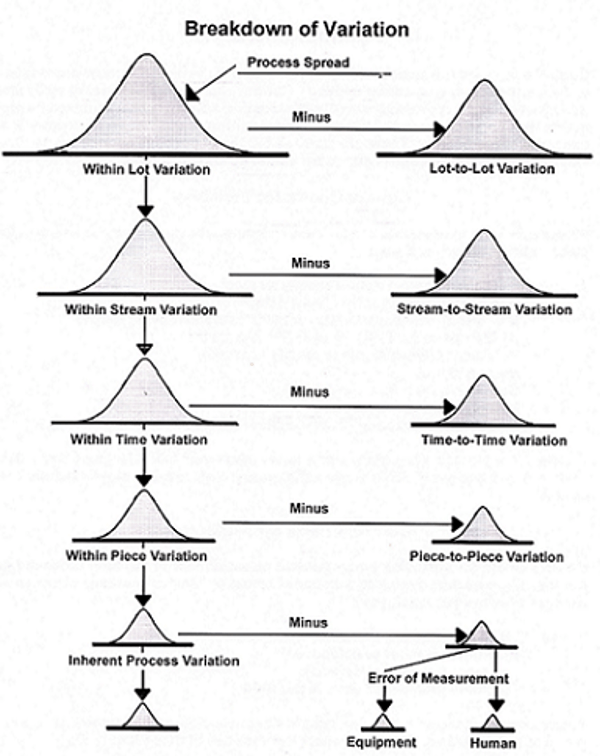### Fundamental concept of Statistical Process Control:

A fundamental concept of statistical process control is that almost every measurable phenomenon is a statistical distribution. In other words, an observed set of data constitutes a sample of the effects of unknown common causes. It follows that, after we have done everything to eliminate special causes of variations, there will still remain a certain
amount of variability exhibiting the state of control.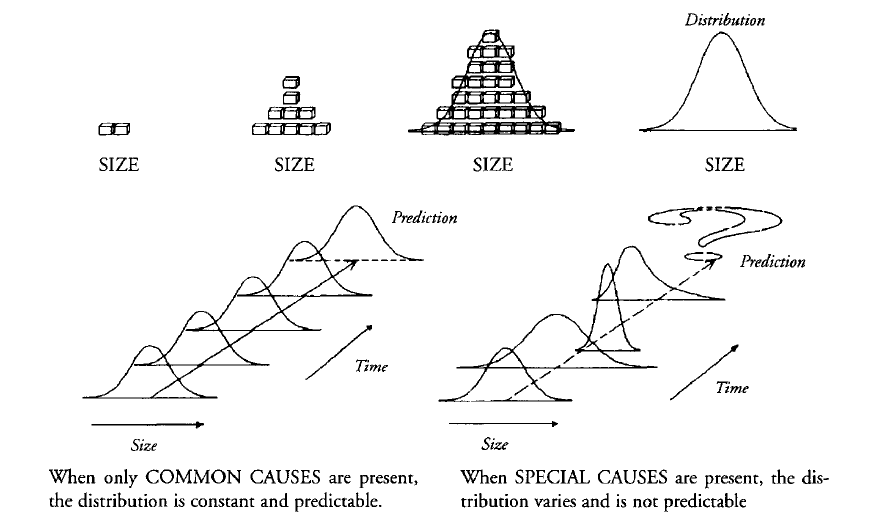Figure above illustrates the relationships between common causes, special causes, and distributions. There are three basic properties of a distribution: location, spread, and shape. The distribution can be characterized by these three parameters. Figure below illustrates these three properties. The location refers to the typical value of the distribution. The spread of the distribution is the amount by which smaller values differ from larger ones. And the shape of a distribution is its pattern—peakedness, symmetry, etc. Note that a given phenomenon may have any of a number of distributions, i.e., the distribution may be bell-shaped, rectangular shaped, etc. In this book we will discuss methods which facilitate the analysis and control of distributions.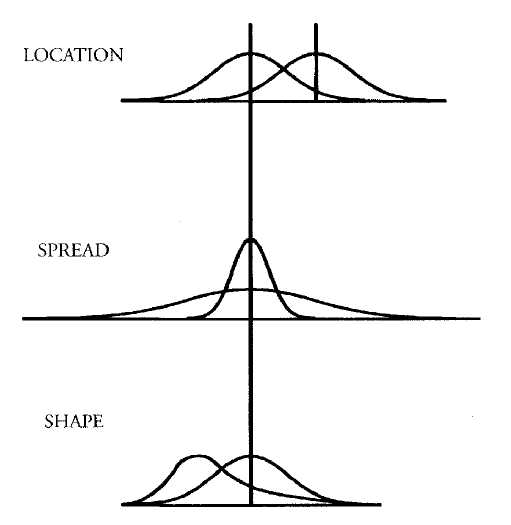The central limit theorem can be stated as follows:
Irrespective of the shape of the distribution of the population or universe, the distribution of average values of samples drawn from that universe will tend toward a normal distribution as the sample size grows without bound.
It can also be shown that the average of sample averages will equal the average of the universe and that the standard deviation of the averages equals the standard deviation of the universe divided by the square root of the sample size. Shewhart performed experiments that showed that small sample sizes were needed to get approximately normal distributions from even wildly non-normal universes. The practical implications of the central limit theorem are immense. Consider that without the central limit theorem effects, we would have to develop a separate statistical model for every non-normal distribution encountered in practice. This would be the only way to determine if the system were exhibiting chance variation. Because of the central limit theorem we can use averages of small samples to evaluate any process using the normal distribution. The central limit theorem is the basis for the most powerful of statistical process control tools, Shewhart control charts.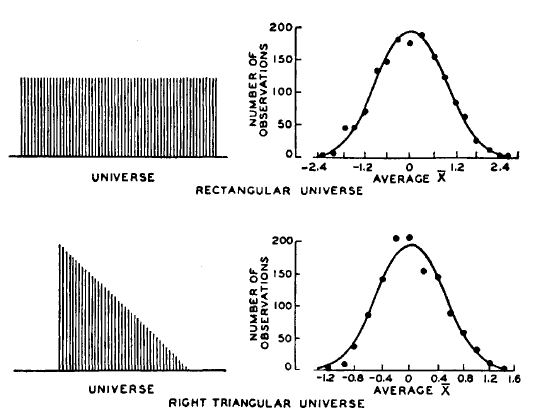If a random variable, x, has mean µ, and finite variance σx2, as n increases,   x̄  approaches a normal distribution with mean µ and variance σ2. Where,and n is the number of observations on which each mean is based.The Central Limit Theorem States:

The sample means i will be more normally distribute around μ than individual readings Xj.  The distribution of sample means approaches normal regardless of the shape of the parent population. The spread in sample means i is less than Xj with standard deviation of i equal to the standard deviation of the population (individuals ) divided by the square root of the sample size S.  is referred to as the standard error of the mean:In the figure a variety of population distribution approaches normally for the sampling distribution of X-bar as n increases. for most distribution but not all a normal sampling distribution is attended with a sample size of 4 or 5.

Types of Control Charts

There are two broad categories of control charts: those for use with continuous data (e.g., measurements) and those for use with attributes data (e.g., counts).

#### Variable Data

Variable charts are based on variable data that can be measured on a continuous scale. For example, weight, volume, temperature or length of stay. These can be measured to as many decimal places as necessary. Individual, average and range charts are used for variable data.

#### Attribute Data

Attribute charts are based on data that can be grouped and counted as present or not. Attribute charts are also called count charts and attribute data is also known as discrete data. Attribute data is measured only with whole numbers. Examples include:

• Acceptable vs. non-acceptable
• Forms completed with errors vs. without errors
• Number of prescriptions with errors vs. without

When constructing attribute control charts, a subgroup is the group of units that were inspected to obtain the number of defects or the number of defective items. Defect and reject (also known as defective) charts are used for attribute data.

Choosing the correct chart for a given a situation is the first step in every analysis. There are actually just a few charts to choose from, and determining the appropriate one requires following some fairly simple rules based on the underlying data. These rules are described in the flowchart below: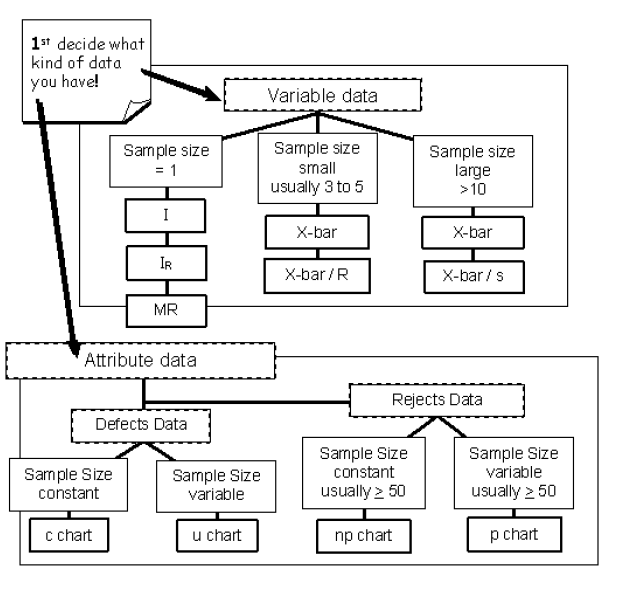### I chart:

The I chart is also referred to as an individual, item, i, or X chart. The X refers to a variable X. Individual Charts plot the process results varying over time. Individual observations are plotted on the I chart, averages are not plotted on this type of chart. Individual charts are used to plot variable data collected chronologically from a process, such as a part’s measurement over time. These charts are especially useful for identifying shifts in the process average. When monitoring a system, it is expected that equal numbers of points will fall above and below the average that is represented by the centerline. Shifts or trends can indicate a change that needs to be investigated. The individual control chart is reserved for situations in which only one measurement is performed each time the data is collected, where it is impractical or impossible to collect a sample of observations. When there are not enough data points to calculate valid control limits, an individual chart functions as a simple run chart.### Average Charts – X-bar Chart

Average charts are made by simply taking the averages of a number of subgroups and plotting the averages on the chart. The average chart is called the X-bar chart because in statistical notation, a bar or line over the variable (X) symbolizes the average of X. “X-bar” is a shorthand way of saying “the average of X”. An X-bar chart is a variable control chart that displays the changes in the average output of a process. The chart reflects either changes over time or changes associated with a categorical data variable. The chart shows how consistent and predictable a process is at achieving the mean. X-bar charts measure variation between subgroups. They are often paired with either Standard Deviation (S) or Range (R) charts, which measure variation within subgroups.

• #### Definition: Variable Data Subgroups

All data in a subgroup has something in common, such as a common time of collection. For example, all data for a particular date, a single shift, or a time of day. Subgroup data can have other factors in common, such as data associated with a particular operator, or data associated with a particular volume of liquid. In Express QC,  this is referred to as a Grouped subgroup and there is a categorical variable that holds the grouping category.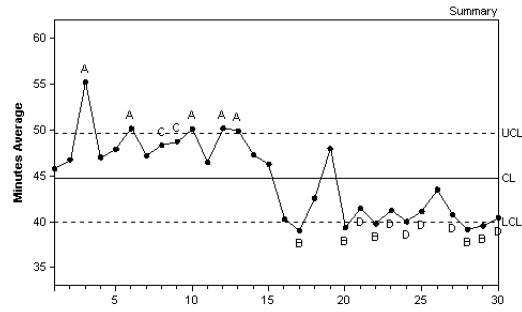### Range Chart – R-Chart

The Range chart can be combined with I charts and X-bar charts. The chart names combine the corresponding chart initials. Range charts measure the variation in the data. An example is the weather report in the newspaper that gives the high and low temperatures each day. The difference between the high and the low is the range for that day.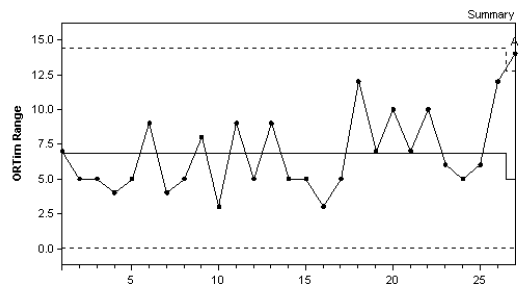### Moving Range Chart – MR Chart

This type of chart displays the moving range of successive observations. A moving range chart can be used when it is impossible or impractical to collect more than a single data point for each subgroup. This chart can be paired with an individual chart, which is then called an Individual Moving Range (IR) chart. An individual chart is used to highlight the changes in a variable from a central value, the mean. The moving range chart displays variability among  measurements based on the difference between one data point and the next.### Individual And Range Charts – IR Charts

This pair of variable control charts is often offered together for quality control analysis. The Individual chart, the upper chart in the figure below, displays changes to the process output over time in relation to the center line which represents the mean. The Moving Range chart, the lower chart in the figure below, analyzes the variation between consecutive observations, which is a measure of process variability.### Average & Range Charts – X-Bar And R Charts

Variable and Range control charts are often displayed together for quality control analysis. The X-bar chart, the upper chart in the figure below, is a graphic representation of the variation among the subgroup averages, the R chart, the lower chart in the figure below, looks at variability within these subgroups. The variation within subgroups is represented by the range (R). The range of values for each subgroup is plotted on the Y-axis of the R chart. The centerline is the average or mean of the range.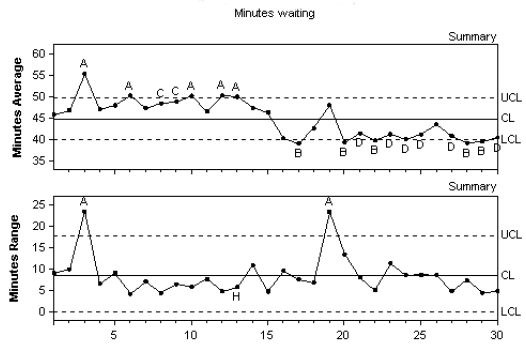#### Example of X bar and R chart

Terms used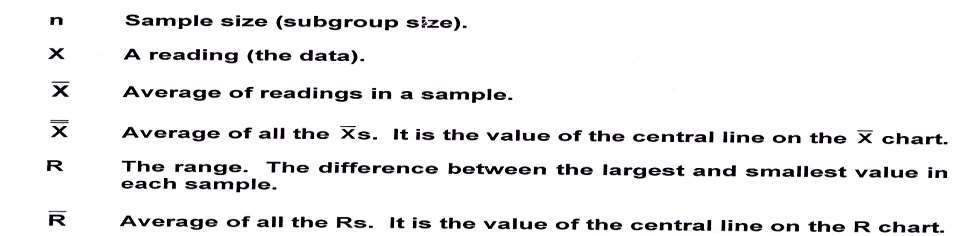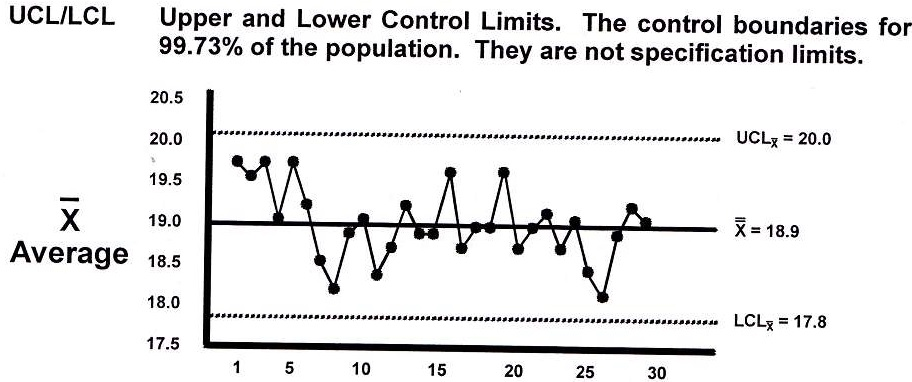### X-Bar Standard Deviation Charts – X-Bar And S Charts

This pair of variable control charts is often displayed together for quality control analysis. The X-bar chart, the upper chart in the figure below, displays the variation in the means between the subgroups. The s chart, the lower chart in the figure below, looks at variability within these subgroups. In this pair of charts, the variation within subgroups is represented by the standard deviation. The standard deviation is plotted on the y-axis, and is a measure of the spread of values for each subgroup. The centerline is the average or mean of these sub-group standard deviations.X-bar and s chart

## X-Bar and Sigma Charts

X-bar( )  and sigma (s) charts are often used for increased sensitivity to variation (especially when larger sample sizes are used). These charts may be more difficult to work with manually than the  – R charts due to the tedious calculation of the sample standard deviation(s). Often, s comes from automated process equipment so the charting process is much easier. The formula is: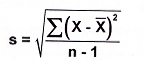Where: Σ means the sum
X the individual measurements
the average
n the sample sizeThe control limits for the sigma (s) chart are calculated using the following formulas.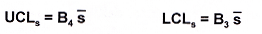s is the average sample standard deviation and is the center line of the sigma chart.Sigma Chart Factors

The estimated standard deviation σ̂ called sigma hat, can be calculated by:c4 Factors

#### Median Control Charts

There are several varieties of median control charts. One type plots only the individual measured data on a single chart. The middle value is circled. Median charts may use an odd number of readings to make the median value more obvious.  Another variety records the data and plots the median value and range on two separate charts. Minimal calculations are needed for each subgroup. The control limits for the median chart are calculated using the same formulas as the – R chart: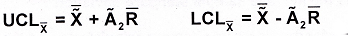The 2values are somewhat different than the 2values for  the – R chart since the median is less efficient and, therefore, exhibits more variation.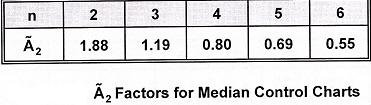The range factors (D3 and D4) and process standard deviation factor (d2) are the same as used for the – R chart.
The specific advantages of a median chart are:

• It is easy to use and requires fewer calculations ,
• It shows the process variation
• It shows both the median and the spread

### MX-bar-MR Charts:

MX-bar – MR (moving average-moving range) charts are a variation of X-bar – R charts where data is less readily available. There are several construction techniques.  An   example for n = 3 is shown below. Control limits are calculated using the X – R formulas and factors.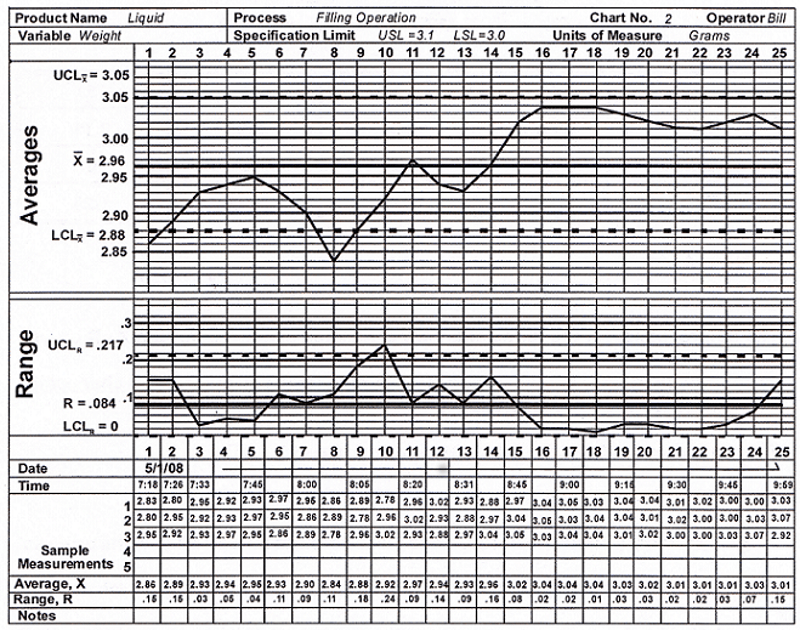## Attribute Data Charts:

Attribute data represents particular characteristics of a product or system that can be counted, not product measurements. They are characteristics that are present or not present. This is known as discrete data, and is measured only with whole numbers.
Examples include:

• Acceptable vs. non-acceptable
• Forms completed with errors vs. without errors
• Number of prescriptions with errors vs. without

Attribute data has another distinctive characteristic. In quality control analysis, this countable data falls into one of two categories:

• Defects data is the number of non-conformities within an item. There is no limit to the number of possible defects. Defects charts count the number of defects in the inspection unit.
• Rejects data or Defective Data where the entire item is judged to conform to product specifications or not. The count for each item is limited to 1or 0. Rejects charts count the number of rejects in a subgroup.

One way to determine what type of data you have is to ask, “Can I count both the occurrences AND non-occurrences of the defective data?” For example, you can count how many forms have errors and how many do not, however you cannot count how many errors were NOT made on the form. If you can count both occurrences and non occurrences, you have rejects data. If the non-occurrences cannot be determined, then you have defects data. For example If you are counting the number of errors made on an insurance form, you have an example of the defects per form. There is no limit to the number of defects that can be counted on each form. If you are counting the total number of forms that had one or more errors, then you have a count of the rejected units. This is either one or zero rejects per unit.

Subgroup size is another important data characteristic to consider in selecting the right type of chart. When constructing attribute control charts, a subgroup is the group of units that were inspected to obtain the number of defects or the number of rejects. To choose the correct chart, you need to determine if the subgroup size is constant or not. If constant, for example 300 forms are processed every day, then you can look at a straight count of the defective occurrences. If the subgroup size changes, you need to look at the percentage or fraction of defective occurrences. For example an organization may have a day in which 500 insurance forms are processed and 50 have errors vs. another day in which only 150 are processed and 20 have errors. If we only look at the count of errors, 50 vs. 20, we would assume the 50 error day was worse. But when considering the total size of the subgroup, 500 vs. 150, we determine that on the first day 10% had errors while the other day 13.3% had errors.

There are four different types of attribute charts. For each type of attribute data, defects and rejects, there is a chart for subgroups of constant size and one for subgroups of varying size.

### c Chart – Constant Subgroup Size

A c chart, or Count chart, is an attribute control chart that displays how the number of defects, or nonconformities, for a process or system is changing over time. The number of defects is collected for the area of opportunity in each subgroup. The area of opportunity can be either a group of units or just one individual unit on which defect counts are performed. The c chart is an indicator of the consistency and predictability of the level of defects in the process. When constructing a c chart, it is important that the area of opportunity for a defect be constant from subgroup to subgroup since the chart shows the total number of defects. When the number of items tested within a subgroup changes, then a u chart should be used, since it shows the number of defects per unit rather than total defects.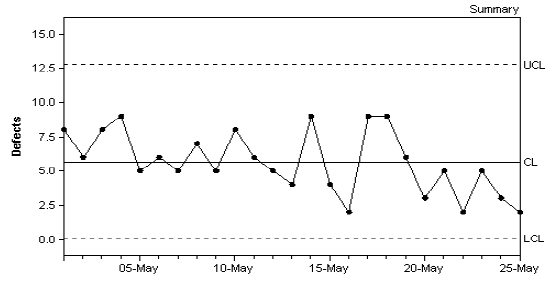#### c Chart Examples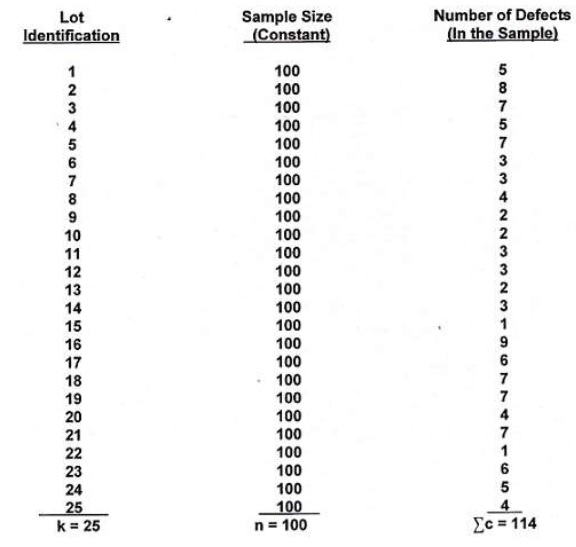### u Chart – Varying Subgroup Size

A u chart (u is for Unit) is an attribute control chart that displays how the frequency of defects, or nonconformities, is changing over time for a process or system. The number of defects is collected for the area of opportunity in each subgroup. The area of opportunity can be either a group of items or just one individual item on which defect counts are performed. The u chart is an indicator of the consistency and predictability of the level of defects in the process. A u chart is appropriate when the area of opportunity for a defect varies from subgroup to subgroup. This can be seen in the shifting UCL and LCL lines that depend on the size of the subgroup. This chart shows the number of defects per unit. When the number of items tested remains the same among all the subgroups, then a c chart should be used since a c chart analyzes total defects rather than the number of defects per unit.### np Chart – Number of Rejects Chart for Constant Subgroup Size

The name of the p chart stands for the Percentage of rejects in a subgroup. The name of the np chart stands for the Number of rejects within a p-type chart. You can also remember it as “not percentage” or “not proportional”.

An np chart is an attribute control chart that displays changes in the number of defective products, rejects or unacceptable outcomes. It is an indicator of the consistency and predictability of the level of defects in the process.  The np chart is only valid as long as your data are collected in subgroups that are the same size. When you have a variable subgroup size, a p chart should be used.#### Np chart Examples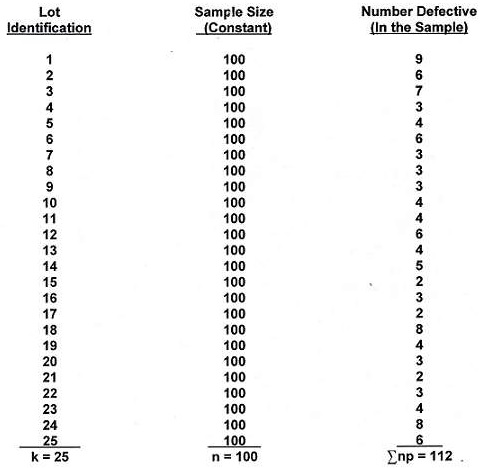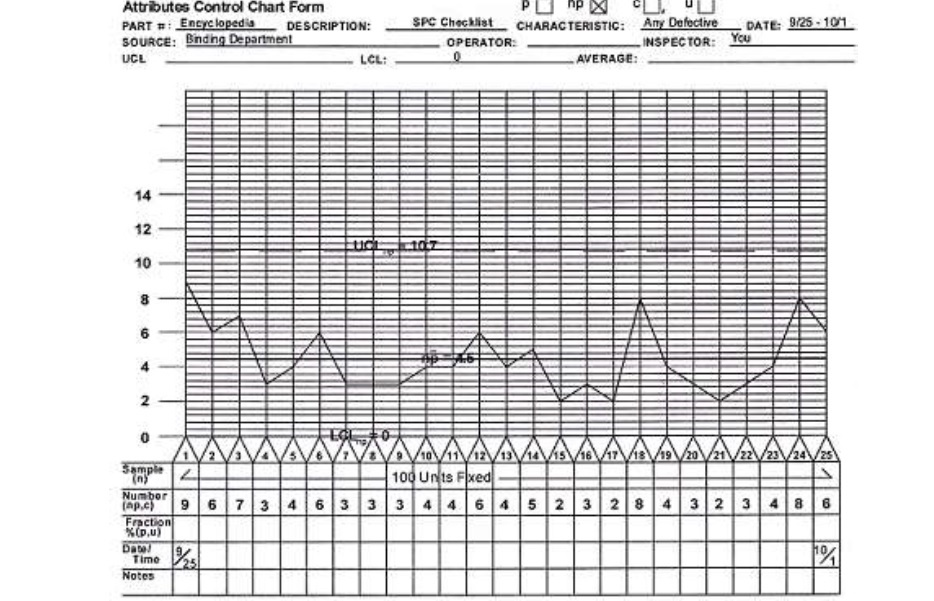### p Chart – Percentage Chart for Varying Subgroup Size

A p chart is an attribute control chart that displays changes in the proportion of defective products, rejects or unacceptable outcomes. It is an indicator of the consistency and predictability of the level of defects in the process. Since a p chart is used when the subgroup size varies, the chart plots the proportion or fraction of items rejected, rather than the number rejected. This is indicated by the shifting UCL and LCL lines that depend on the size of the subgroup. For each subgroup, the proportion rejected is calculated as the number of rejects divided by the number of items inspected. When you have a constant subgroup size, use an np chart instead.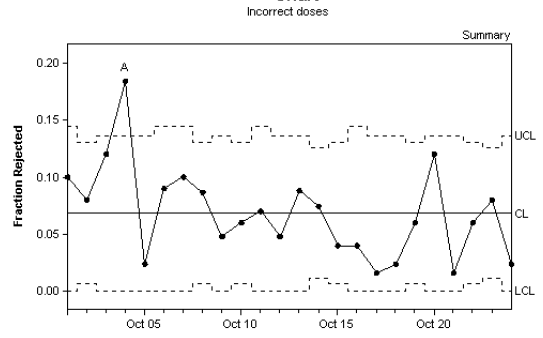#### p chart Example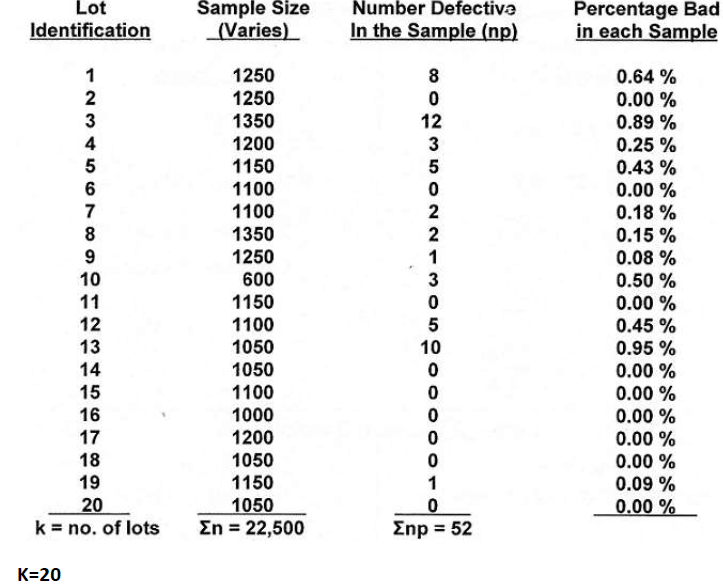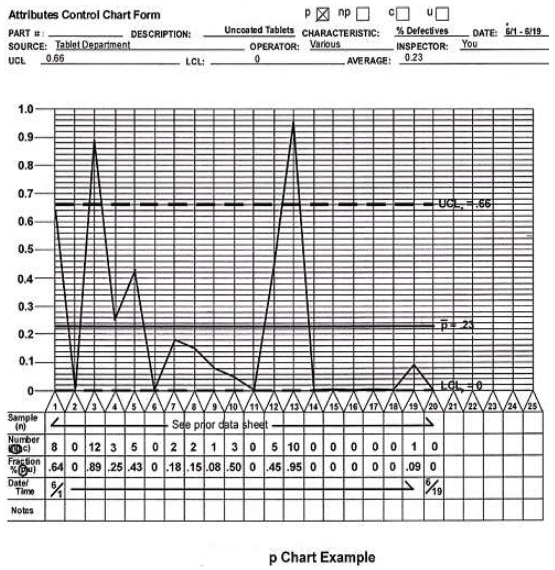### Constructing Control Charts

#### 2. Determine sampling method and plan

• How large a sample can be drawn? Balance the time and cost to collect a sample with the amount of information you will gather.
• As much as possible, obtain the samples under the same technical conditions: the same machine, operator, lot, and so on.
•  Frequency of sampling will depend on whether you are able to discern patterns in the data. Consider hourly, daily, shifts, monthly, annually, lots, and so on. Once the process is “in control,” you might consider reducing the frequency with which you sample.
•  Generally, collect 20–25 groups of samples before calculating the statistics and control limits.
•  Consider using historical data to set a baseline. Make sure samples are random. To establish the inherent variation of a process, allow the process to run untouched, i.e., according to standard procedures.

#### 3. Initiate data collection

• Run the process untouched, and gather sampled data.
• Record data on an appropriate Control Chart sheet or other graph paper. Include any unusual events that occur.

#### 4. Calculate the appropriate statistics

a) If you have attribute data, use the Attribute Data Table, Central Line column.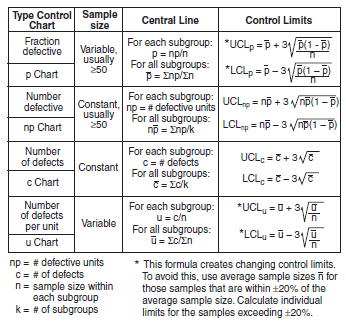b) If you have variable data, use the Variable Data Table, Central Line column.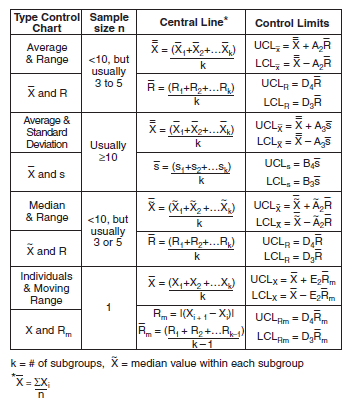##### 5. Calculate the control limits

a) If you have attribute data, use the Attribute Data Table, Control Limits column.

b) If you have variable data, use the Variable Data Table, Control Limits column for the correct formula to use.

• Use the Table of Constants to match the numeric values to the constants in the formulas shown in the Control Limits column of the Variable Data Table. The values you will need to look up will depend on the type of Variable Control Chart you choose and on the size of the sample you have drawn.
• If the Lower Control Limit (LCL) of an Attribute Data Control Chart is a negative number, set the LCL to zero.
• The p and u formulas create changing control limits if the sample sizes vary subgroup to subgroup. To avoid this, use the average sample size, n, for those samples that are within ±20% of the average sample size. Calculate individual limits for the samples exceeding ±20%.

#### 6. Construct the Control Chart(s)

• For Attribute Data Control Charts, construct one chart, plotting each subgroup’s proportion or number defective, number of defects, or defects per unit.
• For Variable Data Control Charts, construct two charts: on the top chart plot each subgroup’s mean, median, or individuals, and on the bottom chart plot each subgroup’s range or standard deviation.
• Draw a solid horizontal line on each chart. This line corresponds to the process average.
• Draw dashed lines for the upper and lower control limits.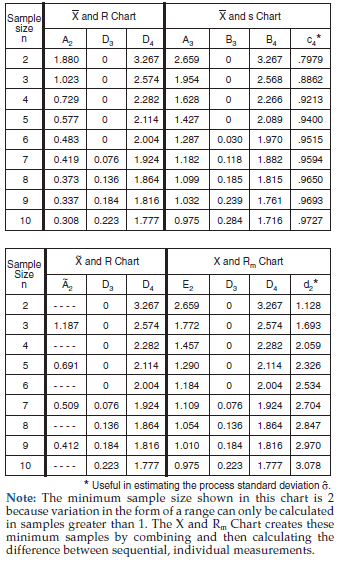### Interpreting Control Charts

• Attribute Data Control Charts are based on one chart. The charts for fraction or number defective, number of defects, or number of defects per unit measure variation between samples. Variable Data Control Charts are based on two charts: the one on top, for averages, medians, and individuals, measures variation between subgroups over time; the chart below, for ranges and standard deviations, measures variation within subgroups over time.
• Determine if the process mean (center line) is where it should be relative to your customer specification or your internal business objective. If not, then it is an indication that your process is not currently capable of meeting the objective.
• Analyze the data relative to the control limits, distinguishing between common causes and special causes. The fluctuation of the points within the limits results from variation inherent in the process. This variation results from common causes within the system, e.g., design, choice of machine, preventive maintenance, and can only be affected by changing that system. However, points outside of the limits or patterns within the limits come from a special cause, e.g., human errors, unplanned events, freak occurrences, that is not part of the way the process normally  operates, or is present because of an unlikely combination of process steps. Special causes must be eliminated before the Control Chart can be used as a monitoring tool. Once this is done, the process will be “in control” and samples can be taken at regular intervals to make sure that the process doesn’t fundamentally change.
• Your process is in “statistical control” if the process is not being affected by special causes. All the points must fall within the control limits, and they must be randomly dispersed about the average line for an in-control system.
• “Control” doesn’t necessarily mean that the product or service will meet your needs. It only means that the process is consistent. Don’t confuse control limits with specification limits—specification limits are related to customer requirements, not process variation.
• Any points outside the control limits, once identified with a cause (or causes), should be removed and the calculations and charts redone. Points within the control limits, but showing indications of trends, shifts, or instability, are also special causes.
• When a Control Chart has been initiated and all special causes removed, continue to plot new data on a new chart, but DO NOT recalculate the control limits. As long as the process does not change, the limits should not be changed. Control limits should be recalculated only when a permanent, desired change has occurred in the process, and only using data after the change occurred.
• Nothing will change just because you charted it! You need to do something. Form a team to investigate.

### Determining if Your Process Is “Out of Control”

A process is said to be “out of control” if either one of these is true:

#### 2. When the Control Chart is divided into zones, as shown below, any of the following points are true: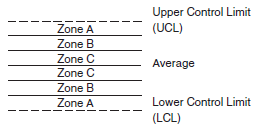a) Two points, out of three consecutive points, are on the same side of the average in Zone A or beyond.
b) Four points, out of five consecutive points, are on the same side of the average in Zone B or beyond.
c) Nine consecutive points are on one side of the average.
d) There are six consecutive points, increasing or decreasing.
e) There are fourteen consecutive points that alternate up and down.
f) There are fifteen consecutive points within Zone C (above and below the average).### AT&T’s Statistical Quality Control Standards

The rules for X-bar charts, Individual charts, Median charts, R charts when the minimum subgroup size is at least 4

1. 1 point above Upper Spec
2. 1 point below Lower Spec
3. 1 point above Zone A
4. 1 point below Zone A
5. 2 of 3 successive points in upper Zone A or beyond
6. 2 of 3 successive points in lower Zone A or beyond
7. 4 of 5 successive points in upper Zone B or beyond
8. 4 of 5 successive points in lower Zone B or beyond
9. 8 points in a row above centerline
10. 8 points in a row below centerline
11. 15 points in a row in Zone C (above and below center)
12. 8 points on both sides of center with 0 in Zone C
13. 14 points in a row alternating up and down
14. 6 points in a row steadily increasing or decreasing

The rules for R charts when the minimum subgroup size is less than 4

1. 1 point above Upper Spec
2. 1 point below Lower Spec
3. 1 point above Zone A
4. 2 successive points in or above upper Zone A
5. 3 successive points in or above upper Zone B
6. 7 successive points in or above upper Zone C
7. 10 successive points in or below lower Zone C
8. 6 successive points in or below lower Zone B
9. 4 successive points in lower Zone A

The rules for s charts, Moving Average charts and Moving Range charts

1. 1 point above Upper Spec
2. 1 point below Lower Spec
3. 1 point above Zone A
4. 1 point below Zone A

The rules for p charts, np charts, c charts and u charts

1. 1 point above Upper Spec
2. 1 point below Lower Spec
3. 1 point above Zone A
4. 1 point below Zone A
5. 9 points in a row above centerline
6. 9 points in a row below centerline
7. 6 points in a row steadily increasing or decreasing
8. 14 points in a row alternating up and down

Back to Home

If you need assistance or have any doubt and need to ask any question  contact us at: preteshbiswas@gmail.com. You can also contribute to this discussion and we shall be happy to publish them. Your comment and suggestion is also welcome.